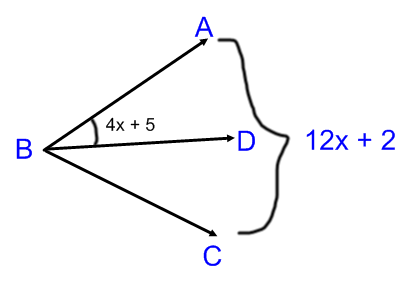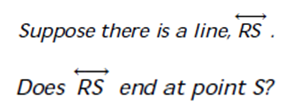GeoA - chapter 1 Skills Maint 3
If AB = 6, What is the length of CD? *
1 point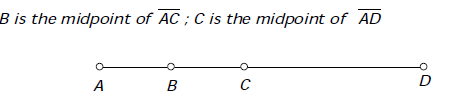Find the length between A and H *
1 pointFind x. *
1 point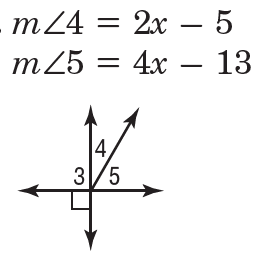Find the distance between the two points *
1 point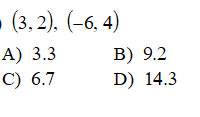<BGA and <AGF are... *
1 point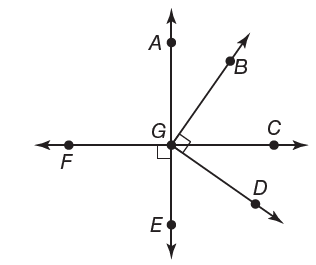Find the distance between the two points *
1 point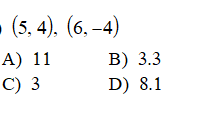what is the vertex of angle 3? *
1 point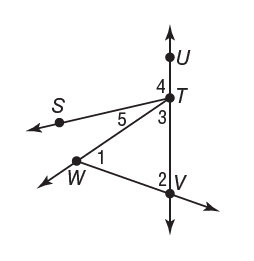Find x. *
1 point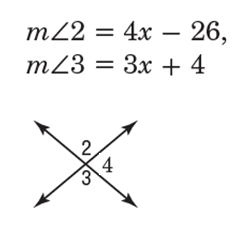Point E is the midpoint of both of these segments. If segment EF = 5x -10, and DE = 45, Find x. *
1 point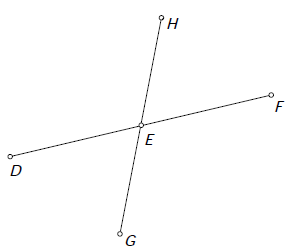Last Name *
There are Two non adjacent complementary angles. THe first angle is labeled 2x + 12 and the second is labeled 6x - 26. Find the value of x. *
1 point
B is the midpoint of segment AC. Find x. *
1 point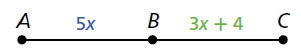Period *
Find angle y *
1 point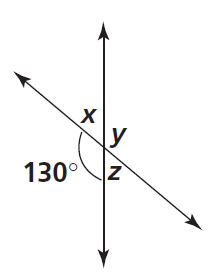Find x. *
1 point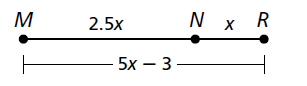Ray BD bisects <ABC. Find x. *
1 point